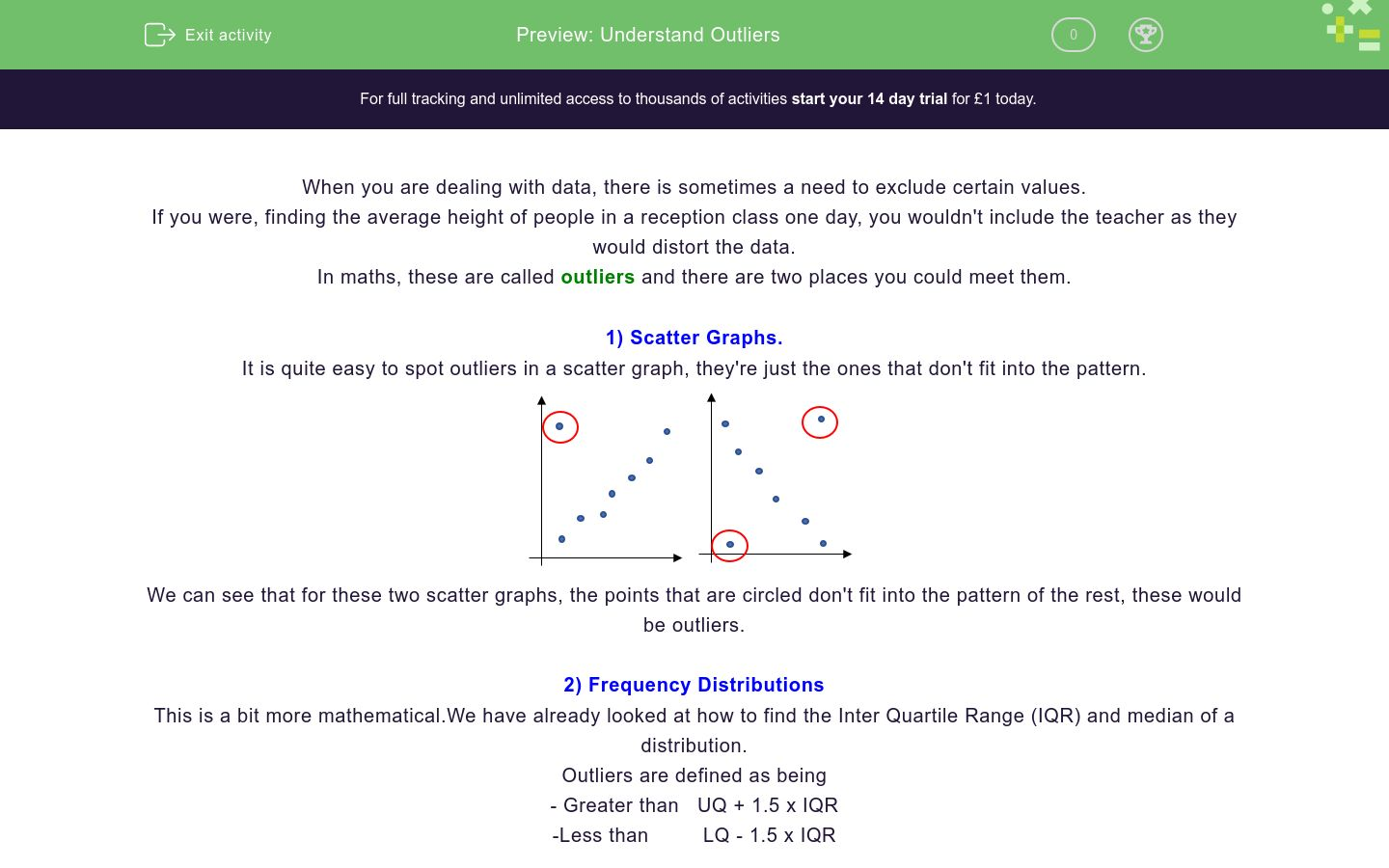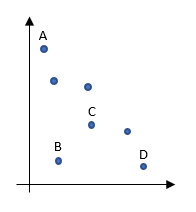# Understand Outliers

In this worksheet, students practise finding the outliers in scatter graphs and frequency distributionsKey stage:  KS 4

GCSE Subjects:   Maths

GCSE Boards:   AQA, Eduqas, Pearson Edexcel, OCR

Curriculum topic:   Statistics

Curriculum subtopic:   Statistics, Analysing Data

Difficulty level:### QUESTION 1 of 10

When you are dealing with data, there is sometimes a need to exclude certain values.

If you were, finding the average height of people in a reception class one day, you wouldn't include the teacher as they would distort the data.

In maths, these are called outliers and there are two places you could meet them.

1) Scatter Graphs.

It is quite easy to spot outliers in a scatter graph, they're just the ones that don't fit into the pattern.We can see that for these two scatter graphs, the points that are circled don't fit into the pattern of the rest, these would be outliers.

2) Frequency Distributions

This is a bit more mathematical.We have already looked at how to find the Inter Quartile Range (IQR) and median of a distribution.

Outliers are defined as being

- Greater than   UQ + 1.5 x IQR

-Less than         LQ - 1.5 x IQR

Example: A data set has a Lower Quartile of 20 and and upper quartile of 28. Find the limits for the outliers.

Step 1: Find the IQR

IQR = UQ - LQ = 28 - 20 = 8

Step 2: Find 1.5 x IQR

1.5 x IQR = 1.5 x 8 = 12

Step 3: Find the limits for the outliers

UQ + 1.5 x IQR = 28 + 12 = 40

LQ - 1.5 x IQR = 20 - 12 = 8

This means that any numbers below 8 or above 40 in the distribution are outliers.

An outlier on a scatter graph is...

An outlier is any value that will...

To find the outliers for a frequency distribution, we...

Which of these points can be considered as outliers?A

B

C

D

Which of these points can be considered as outliers?A

B

C

D

I have an IQR of 30. What would I need to add to the UQ and subtract from the LQ to find the limits of the outliers?

I have an upper quartile of 12 and a lower quartile of 8.

What would be the limits of the outliers?

 Value Greater than Less than

I have an upper quartile of 20 and a lower quartile of 10.

What would be the limits of the outliers?

 Value Greater than Less than

I have an upper quartile of 20 and a lower quartile of 14.

Which of these numbers would be outliers for this data set

1

4

5

20

25

30

I have an upper quartile of 8 and a lower quartile of 6.

Would 2 be an outlier for this data?

Yes

No

• Question 1

An outlier on a scatter graph is...

EDDIE SAYS
When we are dealing with a scatter graph, we are looking for a pattern. Outliers are ones that don't fit into the pattern.
• Question 2

An outlier is any value that will...

EDDIE SAYS
An outlier will mess about with the data and make some of the calculations you do not work. We call this distorting the data
• Question 3

To find the outliers for a frequency distribution, we...

EDDIE SAYS
Why 1.5? Why not, the number had to be set somewhere.
• Question 4

Which of these points can be considered as outliers?B
EDDIE SAYS
An outlier is one that doesn\'t fit into the general pattern. This is a negative correlation and there is only one that fits the definition.
• Question 5

Which of these points can be considered as outliers?B
D
EDDIE SAYS
An outlier is one that doesn't fit into the general pattern. This is a positive correlation and there are two that fit the definition.
• Question 6

I have an IQR of 30. What would I need to add to the UQ and subtract from the LQ to find the limits of the outliers?

45
EDDIE SAYS
Remember the rule for finding the limits Limits = UQ + 1.5 x IQR
• Question 7

I have an upper quartile of 12 and a lower quartile of 8.

What would be the limits of the outliers?

 Value Greater than Less than
EDDIE SAYS
If we find the IQR we get 12 - 8 = 4 Finding 1.5 x IQR gives 1.5 x 4 = 6 We then just add this onto the UQ and subtract it from the LQ
• Question 8

I have an upper quartile of 20 and a lower quartile of 10.

What would be the limits of the outliers?

 Value Greater than Less than
EDDIE SAYS
If we find the IQR we get 20 - 10 = 10 Finding 1.5 x IQR gives 1.5 x 10 = 15 We then just add this onto the UQ and subtract it from the LQ
• Question 9

I have an upper quartile of 20 and a lower quartile of 14.

Which of these numbers would be outliers for this data set

1
4
30
EDDIE SAYS
If we find the IQR we get 23 - 14 = 6 Finding 1.5 x IQR gives 1.5 x 6 = 9 We can use this to find the outliers will be anything below 5 and above 29. Remember that is has to be below 5 to count.
• Question 10

I have an upper quartile of 8 and a lower quartile of 6.

Would 2 be an outlier for this data?

Yes
EDDIE SAYS
If we find the IQR we get 8 - 6 = 2 Finding 1.5 x IQR gives 1.5 x 2 = 3 We can use this to find the outliers will be anything below 3 and above 11
---- OR ----

Sign up for a £1 trial so you can track and measure your child's progress on this activity.

### What is EdPlace?

We're your National Curriculum aligned online education content provider helping each child succeed in English, maths and science from year 1 to GCSE. With an EdPlace account you’ll be able to track and measure progress, helping each child achieve their best. We build confidence and attainment by personalising each child’s learning at a level that suits them.

Get started